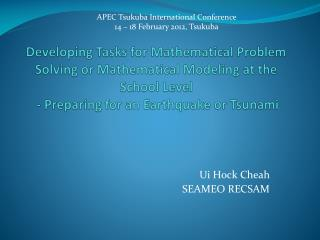DownloadDownload PresentationUi Hock Cheah SEAMEO RECSAM

# Ui Hock Cheah SEAMEO RECSAM

Download Presentation## Ui Hock Cheah SEAMEO RECSAM

- - - - - - - - - - - - - - - - - - - - - - - - - - - E N D - - - - - - - - - - - - - - - - - - - - - - - - - - -
##### Presentation Transcript

1. APEC Tsukuba International Conference 14 – 18 February 2012, Tsukuba Developing Tasks for Mathematical Problem Solving or Mathematical Modeling at the School Level - Preparing for an Earthquake or Tsunami Ui Hock Cheah SEAMEO RECSAM

2. Real Problem Solving and Mathematical Modelling The important features of the task: • The problem: problem must be real and connected to the students • Aim is for the students to transforming the real-life problem into a mathematical problem

3. Real World Mathematical World Real World Problem Mathematical Problem Real World Situation Mathematical Solution and a Mathematical Model

4. Outline of the Mathematical Modelling Process for the Students Real life situation Formulate a mathematical problem Identify the actual problem Set up a model Find a mathematical solution Compare with the original solution Interpret the solution Write the solution/ report

5. Challenges of Math Modelling in school • Selecting tasks that are relevant and appropriate • Helping students connect with the task • Helping students look at the real world problem with mathematical eyes • Helping students pose mathematical questions from a real world situation • Moving from specific to the general solution

6. Using Problem-based learning (PBL) to overcome some of the challenges • Using scenarios to connect students to the real world • Focusing on what the students know from the scenarios and what they need to know • Allowing students to pose and frame their own mathematical questions • Allowing students to plan strategies to solve the problem

7. Exemplar • Objective: Using the scenario of preparedness for natural disasters to lead students to examine estimates, probability in preparing an evacuation center • School level: Grade 8 • Lesson plan will covered over 2 lessons • A video on tsunami can be used to introduce the lesson

8. Scenario 1 You are the Director of Planning in a town along the South coast of Japan. The population of the town is about 20,000 people. The Government is concerned about the safety of the people in case of natural disasters. • What do you know? • What do you need to know?

9. Scenario 2 The Minister of Education asks you to plan an evacuation center for the town which will be used during an earthquake or a Tsunami. Your task is to lead a team of planners to design the evacuation centre and submit it to the government

10. What do you know? • What do you need to know? In your group, write down the questions you want to ask. What other information do you need? • What mathematics questions can you ask? • What information do you need to answer the questions?